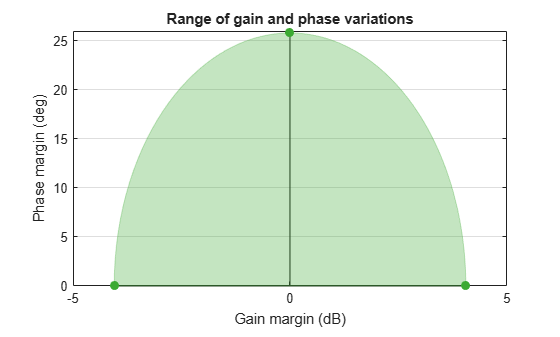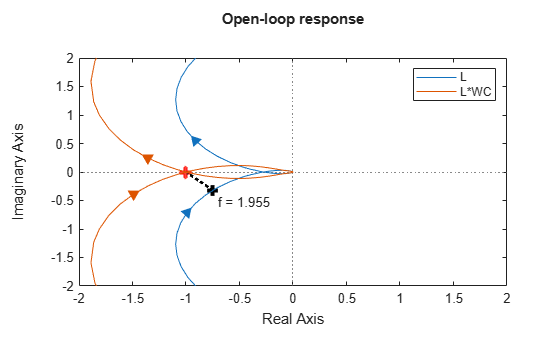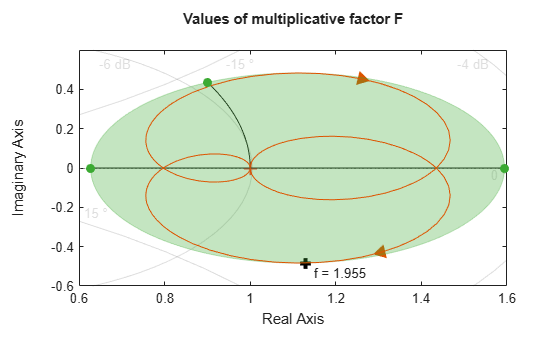# Disk Margin and Smallest Destabilizing Perturbation

This example shows how to interpret the `WorstPerturbation` field in the structure returned by `diskmargin`, the smallest gain and phase variation that results in closed-loop instability.

### Disk Margin as Range of Allowable Gain and Phase Variations

Compute the disk margins of a SISO feedback loop with open-loop response `L`.

```L = tf(25,[1 10 10 10]); DM = diskmargin(L);```

The disk-based margins define a range of "safe" gain and phase variations for which the feedback loop remains stable. The `diskmarginplot` command lets you visualize this range as a region in the gain-phase plane. As long as gain and phase variations stay within the shaded region, the closed-loop system `feedback(L,1)` remains stable.

`diskmarginplot(DM.GainMargin)``diskmargin` models gain and phase variations as a complex-valued multiplicative factor `F` applied to the nominal loop transfer `L`. The set of `F` values is a disk whose intersection with the real axis is the interval `DM.GainMargin`. (See Stability Analysis Using Disk Margins.) `diskmarginplot` can also plot the `F` disk.

`diskmarginplot(DM.GainMargin,'disk')``diskmargin` also computes the smallest variation that destabilizes the feedback loop, returned in the field `DM.WorstPerturbation`. This variation is returned as a state-space model that realizes the destabilizing gain and phase variation. When you multiply L by this perturbation, the resulting closed-loop system has an undamped pole at the frequency returned in `DM.Frequency`.

```WC = DM.WorstPerturbation; CL = feedback(L*WC,1); damp(CL)```
``` Pole Damping Frequency Time Constant (rad/seconds) (seconds) -1.49e+00 + 7.93e-01i 8.83e-01 1.69e+00 6.70e-01 -1.49e+00 - 7.93e-01i 8.83e-01 1.69e+00 6.70e-01 3.89e-16 + 1.96e+00i -1.99e-16 1.96e+00 -2.57e+15 3.89e-16 - 1.96e+00i -1.99e-16 1.96e+00 -2.57e+15 -4.19e+00 1.00e+00 4.19e+00 2.39e-01 -9.46e+00 1.00e+00 9.46e+00 1.06e-01 ```

Verify that the gain and phase variation of the destabilizing perturbation mark a boundary point for the range of "safe" gain and phase variations. To do so, compute the gain and phase of `WC` at `DM.Frequency`.

```hWC = freqresp(WC,DM.Frequency); GM = mag2db(abs(hWC))```
```GM = 1.7832 ```
`PM = 180/pi * abs(angle(hWC))`
```PM = 23.1695 ```
```diskmarginplot(DM.GainMargin) line(GM,PM,'Color','k','Marker','+','MarkerSize',8,'LineWidth',3,'HandleVisibility','off') text(GM+.1,PM+1,sprintf('Gain and phase of WC\n at f = %.5g',DM.Frequency))```### Nyquist Interpretation

The statement that the perturbation `WC` drives the closed-loop system unstable is equivalent to saying that the Nyquist plot of `L*WC` touches the critical point at the frequency `DM.Frequency`. (See Stability Analysis Using Disk Margins.) The following plot shows the Nyquist plots of `L` and `L*WC`. The crosses on each plot mark the response at `DM.Frequency`, and confirm that the response of `L*WC` is –1 at this frequency.

```figure(2), clf hL = freqresp(L,DM.Frequency); nyquist(L,L*WC), title('Open-loop response') legend('L','L*WC') axis([-2 2 -2 2]) line(-1,0,'Color','r','Marker','+','MarkerSize',8,... 'LineWidth',3,'HandleVisibility','off') line(real(hL),imag(hL),'Color','k','Marker','+',... 'MarkerSize',8,'LineWidth',3,'HandleVisibility','off') text(real(hL)+0.05,imag(hL)-0.2,sprintf('f = %.5g',DM.Frequency)) line([real(hL) -1],[imag(hL) 0],'Color','k','LineStyle',':',... 'LineWidth',2,'HandleVisibility','off')```The perturbation `WC` is dynamic and its Nyquist plot hugs the boundary of the disk of `F` values. The point of contact is the frequency `DM.Frequency` where the disk margin is weakest. The following plot uses `diskmarginplot` to render the disk of allowable gain and phase variations on the Nyquist plane, superimposing the response of the perturbation `WC`. The black cross again marks the response at `DM.Frequency`.

```hWC = freqresp(WC,DM.Frequency); diskmarginplot(DM.GainMargin,'disk') hold on nyquist(WC) hold off axis([0.6 1.6 -0.6 0.6]) line(real(hWC),imag(hWC),'Color','k','Marker','+',... 'MarkerSize',8,'LineWidth',3,'HandleVisibility','off') text(real(hWC)+0.02,imag(hWC)-0.05,sprintf('f = %.5g',DM.Frequency))```Equivalently, this frequency is where the Nyquist plot of `L` touches the exclusion region associated with the disk margins `DM`. The following plot shows the disk of variations with the Nyquist plot of `L`. The black cross marks the response of `L` at `DM.Frequency`.

```diskmarginplot(DM.GainMargin,'nyquist') hold on nyquist(L) hold off axis([-2 0 -1 1]) line(real(hL),-imag(hL),'Color','k','Marker','+',... 'MarkerSize',8,'LineWidth',3,'HandleVisibility','off') text(real(hL)+0.05,-imag(hL)+0.05,sprintf('f = %.5g',DM.Frequency))```Thus, the disk `F` represents a region in the Nyquist plane that the response of `L` cannot enter while preserving closed-loop stability. At the critical frequency `DM.Frequency`, the frequency at which the gain and phase margins are smallest, the Nyquist plot of `L` just touches the disk.